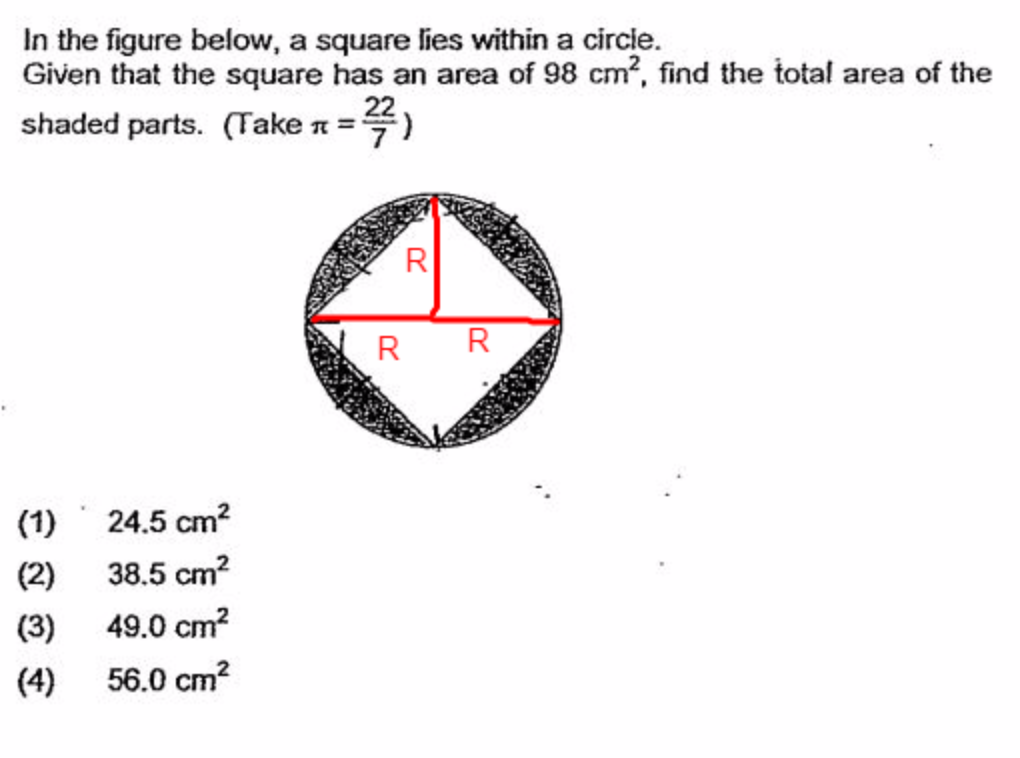# QuestionIf you cut the square into half, it is made of 2 triangles with base =2R and height =R

Area of one of the triangle = 98/2 = 49 cm2

1/2 (2R) .R = 49 cm2

R2 = 49

R =7 cm

Area of circle = 22/7 X 7 X7 = 154 cm2

Shaded parts = 154 -98 = 60   56cm2

Edit note: careless mistake with the calculator. Correction made. Answer is 56 cm2 and not 60.